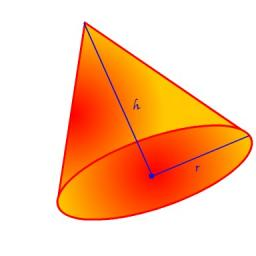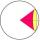# Rotary cone

Rotary cone whose height is equal to the circumference of the base, has a volume 229 cm3.

Calculate the radius of the base circle and height of the cone.

Correct result:

r =  3.26 cm
h =  20.51 cm

#### Solution:We would be pleased if you find an error in the word problem, spelling mistakes, or inaccuracies and send it to us. Thank you!#### You need to know the following knowledge to solve this word math problem:

We encourage you to watch this tutorial video on this math problem:

## Next similar math problems:

• Rotary coneThe volume of the rotation of the cone is 472 cm3 and angle between the side of the cone and base angle is 70°. Calculate lateral surface area of this cone.
• ConeCircular cone with height h = 29 dm and base radius r = 3 dm slice plane parallel to the base. Calculate distance of the cone vertex from this plane, if solids have the same volume.
• SphereIntersect between plane and a sphere is a circle with a radius of 60 mm. Cone whose base is this circle and whose apex is at the center of the sphere has a height of 34 mm. Calculate the surface area and volume of a sphere.
• ConeCircular cone of height 15 cm and volume 5699 cm3 is at one-third of the height (measured from the bottom) cut by a plane parallel to the base. Calculate the radius and circumference of the circular cut.
• Vertical prismThe base of vertical prism is a right triangle with leg a = 5 cm and a hypotenuse c = 13 cm. The height of the prism is equal to the circumference of the base. Calculate the surface area and volume of the prism
• Height as diameter of baseThe rotary cylinder has a height equal to the base diameter and the surface of 471 cm2. Calculate the volume of a cylinder.
• Equilateral cylinderEquilateral cylinder (height = base diameter; h = 2r) has a volume of V = 199 cm3 . Calculate the surface area of the cylinder.
• Lateral surface areaThe ratio of the area of the base of the rotary cone to its lateral surface area is 3: 5. Calculate the surface and volume of the cone, if its height v = 4 cm.
• CylinderCalculate the dimensions of rotating cylindrical container with volume 2 l, if height of container is equal to the diameter of the base.
• The diagram 2The diagram shows a cone with slant height 10.5cm. If the curved surface area of the cone is 115.5 cm2. Calculate correct to 3 significant figures: *Base Radius *Height *Volume of the cone
• Triangular pyramidCalculate the volume and surface area of a regular triangular pyramid whose height is equal to the length of the base edges 10 cm.
• Cylinder surface areaVolume of a cylinder whose height is equal to the radius of the base is 678.5 dm3. Calculate its surface area.
• Diameter = heightThe surface of the cylinder, the height of which is equal to the diameter of the base, is 4239 cm square. Calculate the cylinder volume.
• Tin with oilTin with oil has the shape of a rotating cylinder whose height is equal to the diameter of its base. Canned surface is 1884 cm2. Calculate how many liters of oil is in the tin.
• Slant heightThe slant height of cone is 5cm and the radius of its base is 3cm, find the volume of the cone
• Concrete blockDetermine the volume of concrete block whose one edge of the base has a length 3 meters, body diagonal is 13 meters and its height is 12 meters.
• Rhombus baseCalculate the volume and surface area of prisms whose base is a rhombus with diagonals u1 = 12 cm and u2 = 15 cm. Prism height is twice base edge length.